2017-11-22

Marginal Likelihood in Python and PyMC3

(Long post ahead, so if you would rather play with the code, the original Jupyter Notebook could be found on Gist).

This blog post is based on the paper reading of A Tutorial on Bridge Sampling, which gives an excellent review of the computation of marginal likelihood, and also an introduction of Bridge sampling. Here I will replicate these computations in Python and PyMC3, implementing especially the Bridge sampling technique. An R implementation of Bridge sampling is available on Github with the paper.

## Background

The marginal likelihood is defined as follow:

$$p(y \mid \text{Model}) = \int p(y \mid \theta, \text{Model}) p(\theta \mid \text{Model}) d \theta$$ where $y$ is the observed data, and $\text{Model}$ is the model/parameterization. If you interprete the marginal likelihood as a weighted average of the likelihood of the data given the prior $p(\theta \mid \text{Model})$, you can rewrite marginal likelihood as an expectation:

$$p(y \mid \text{Model}) = \mathbb{E}_{prior} [ p(y \mid \theta, \text{Model}) ]$$

The upside of thinking regarding Expectation is that, using Monte Carlo simulation, we can think of simulating lots of samples (from the prior) and take their average.

!Warning!: Marginal likelihood (and Bayes Factor) is extremely sensitive to your model parameterisation (particularly the priors). You should be aware of which part of the model the marginal likelihood is sensitive to, and have a clear justification of these parts. Otherwise, Bayes Factors are too easy to game and you will most likely fool yourself.

## The Running Example: The Beta-Binomial Model¶

$$\theta \sim \text{Beta}(1,1)$$ $$k \sim \text{Binomial}(\theta,n)$$

With a Conjugate prior, this becomes a Beta-Binomial model which the analytical solution is aviable:
Posterior $$\theta \mid n, k \sim \text{Beta}(k+1, n-k+1)$$ and the marginal $$p (k \mid n) = \int^1_0 p (k \mid \theta, n) p (\theta) d (\theta) = \dfrac{1}{n+1}$$

The running example is based on the R code accompany with the Tutorial paper on Bridge Sampling. More details please see the paper.

# Observed Data
k = 2
n = 10
# Prior parameters for Beta
alpha = beta = 1


## The Analytical Solution¶

# Exact Calculation
m_l = 1/(n + 1)
print('The analytical solution of the marginal likelihood is %.5f'%(m_l))

The analytical solution of the marginal likelihood is 0.09091


x = np.linspace(0.0, 1.0, 1000)
f = lambda a, b : st.beta.pdf(x, a, b)

fig, ax = plt.subplots()
plot_pdf = lambda a, b : ax.plot(x, f(a,b), label=r'$\alpha$={0}, $\beta$={1}'.format(a,b))
plot_pdf(alpha, beta)
plot_pdf(k+alpha, n-k+beta)
plt.legend(loc='upper center', frameon=False)
ax.set(xlim=[0,1], ylim=[0,3.5], xlabel='x', ylabel='pdf')
plt.show()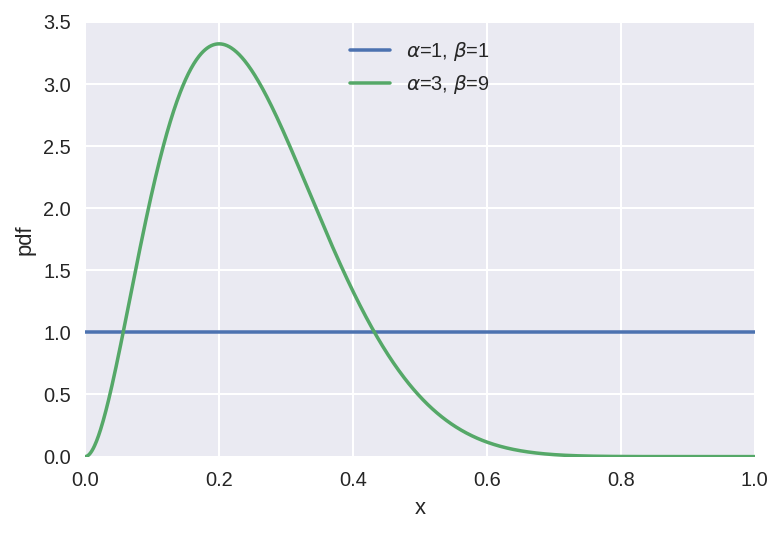## The Naive Monte Carlo Estimator¶

AKA, sample from the prior and hope for the best. It is quite wasteful because quite a lot of samples will contribute little to the integral.

N=12
prior_samples = st.beta.rvs(alpha, beta, size=N)

fig, ax = plt.subplots()
betap = st.beta.pdf(prior_samples, alpha, beta)
ax.plot(prior_samples, betap, 'ko')
ax.plot([prior_samples, prior_samples],
[np.zeros_like(betap), betap], 'k--', alpha=.5)

plot_pdf(alpha, beta)
plot_pdf(k+alpha, n-k+beta)
plt.legend(loc='upper center', frameon=False)
ax.set(xlim=[0, 1], ylim=[0, 3.5], xlabel='x', ylabel='pdf')
plt.show()

m_l_n = st.binom.pmf(k, n, p=prior_samples).mean()
print('The Naive Monte Carlo Estimation is %.5f'%(m_l_n))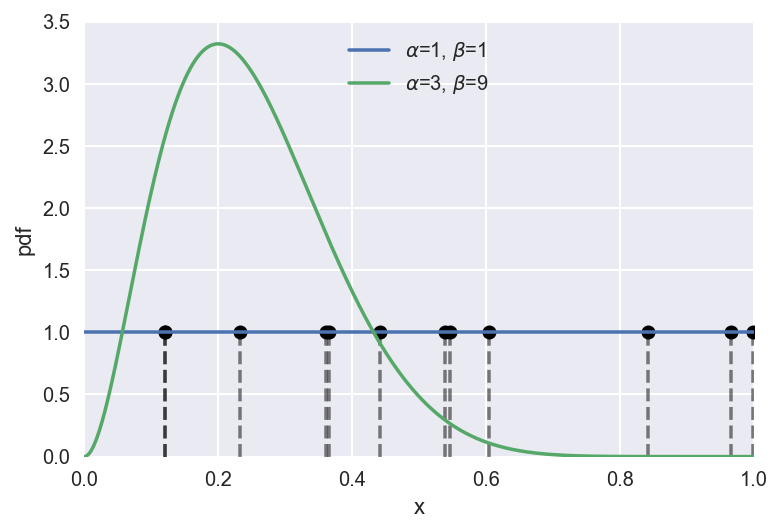The Naive Monte Carlo Estimation is 0.10193


Naive Monte Carlo Estimation using PyMC3 model: first set up a PyMC3 model

# Set up PyMC3 model
with pm.Model() as BB:
p = pm.Beta('p', alpha=1., beta=1., transform=None)
obs = pm.Binomial('obs', p=p, n=n, observed=k)


I then get the log-probability function from PyMC3 model. I should point out that when I am defining the model above, I set the transform=None for the Beta distribution. In general, PyMC3 transformed the bounded parameters to Real line, however, doing so here the logp function will evaluate on the transformed input.

logp_func = BB.logp_array


prior_samples = p.random(size=N)

fig, ax = plt.subplots()
betap = st.beta.pdf(prior_samples, alpha, beta)
ax.plot(prior_samples, betap, 'ko')
ax.plot([prior_samples, prior_samples],
[np.zeros_like(betap), betap], 'k--', alpha=.5)
plot_pdf(alpha, beta)
plot_pdf(k+alpha, n-k+beta)
plt.legend(loc='upper center', frameon=False)
ax.set(xlim=[0,1], ylim=[0,3.5], xlabel='x', ylabel='pdf')
plt.show()

m_l_n = np.exp(np.array([logp_func(p) for p in prior_samples])).mean()
print('The Naive Monte Carlo Estimation is %.5f'%(m_l_n))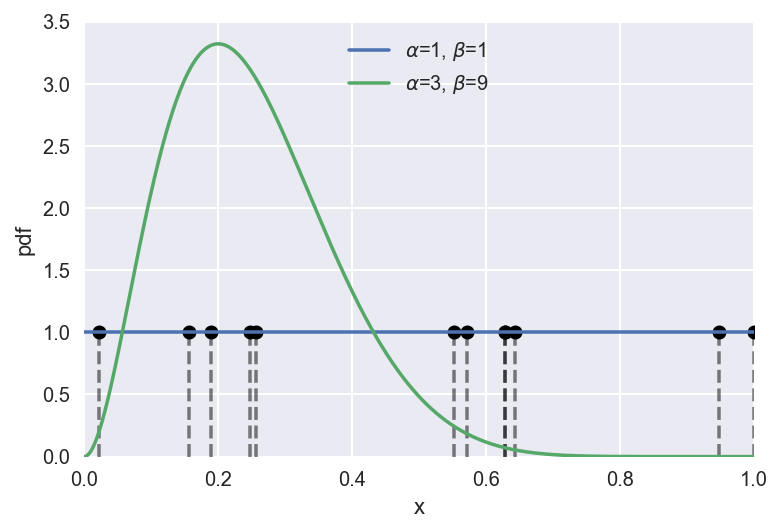The Naive Monte Carlo Estimation is 0.10145


## The Importance Sampling Estimator¶

The idea here is to sample from an Importance density to reduce the variance of the estimation. The key to Importance sampling usually is choosing an importance density, which I will not go into detail here and just follow the paper to construct a mixture distribution that has fatter tail than the posterior.

Some helper function for creating the Importance density:

# helper functions
def posterior_ab(post_samples):
# use properties from the posterior in order to create g
mu_post = np.mean(post_samples)
var_post = np.var(post_samples)

# use method of moments to obtain best fitting beta distribution
a_g = mu_post*(mu_post*(1 - mu_post)/var_post - 1)
b_g = (1 - mu_post)*(mu_post*(1 - mu_post)/var_post - 1)
return a_g, b_g

# mixture distribution
def betamix_pdf(x, w, a_g, b_g):
return w*st.beta.pdf(x, alpha, beta) + (1-w)*st.beta.pdf(x, a_g, b_g)

# mixture samples
def betamix_rvs(N, w, a_g, b_g):
mix_ind = np.random.choice(2, N, p=[w, 1-w])
betamix_rv = np.hstack((st.beta.rvs(alpha, beta, size=(N, 1)),
st.beta.rvs(a_g, b_g, size=(N, 1))))
return betamix_rv[range(N), mix_ind]


w = .3
# Draw from the posterior and create a Important Density g_IS(theta)
post_samples = st.beta.rvs(k+alpha, n-k+beta, size=N)

a_g, b_g = posterior_ab(post_samples)
g_samples = betamix_rvs(N, w, a_g, b_g)
betap = betamix_pdf(g_samples, w, a_g, b_g)

fig, ax = plt.subplots()
ax.plot(g_samples, betap, 'ko')
ax.plot([g_samples, g_samples],
[np.zeros_like(betap), betap], 'k--', alpha=.5)
ax.plot(x, betamix_pdf(x, w, a_g, b_g))
plot_pdf(k+alpha, n-k+beta)
plt.legend(loc='upper center', frameon=False)
ax.set(xlim=[0,1], ylim=[0,3.5], xlabel='x', ylabel='pdf')
plt.show()

m_l_i = (st.binom.pmf(k, n, p=g_samples)*st.beta.pdf(g_samples, alpha, beta)/
betamix_pdf(g_samples, w, a_g, b_g)).mean()
print('The Importance Sampling Estimation is %.5f'%(m_l_i))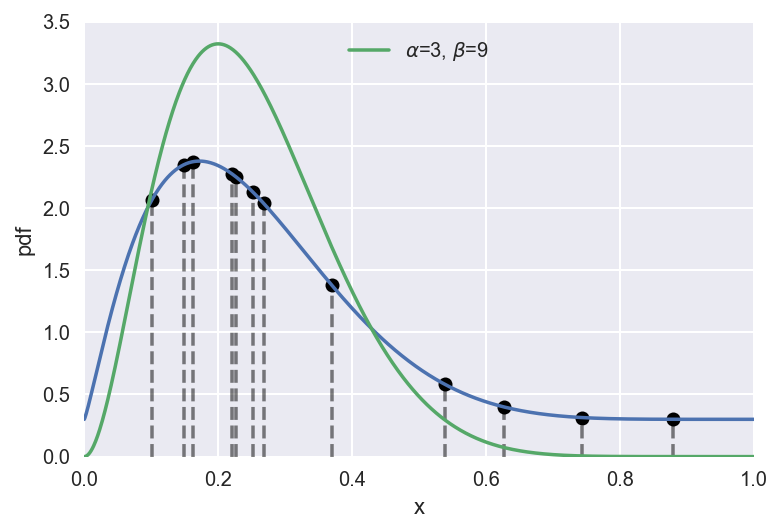The Importance Sampling Estimation is 0.08610


Using PyMC3, this time take samples from the posterior, and use the posterior sample to construct the Importance density:

# Set up PyMC3 model
with pm.Model() as BB:
p = pm.Beta('p', alpha=1., beta=1.)
obs = pm.Binomial('obs', p=p, n=n, observed=k)
trace = pm.sample()

Auto-assigning NUTS sampler...
100%|██████████| 1000/1000 [00:01<00:00, 662.90it/s]


post_samples = trace['p']

a_g, b_g = posterior_ab(post_samples)
g_samples = betamix_rvs(N, w, a_g, b_g)
betap = betamix_pdf(g_samples, w, a_g, b_g)

fig, ax = plt.subplots()
ax.plot(g_samples, betap, 'ko')
ax.plot([g_samples, g_samples],
[np.zeros_like(betap), betap], 'k--', alpha=.5)
ax.plot(x, betamix_pdf(x, w, a_g, b_g))
plot_pdf(k+alpha, n-k+beta)
plt.legend(loc='upper center', frameon=False)
ax.set(xlim=[0,1], ylim=[0,3.5], xlabel='x', ylabel='pdf')
plt.show()

m_l_i = (st.binom.pmf(k, n, p=g_samples)*st.beta.pdf(g_samples, alpha, beta)/
betamix_pdf(g_samples, w, a_g, b_g)).mean()
print('The Importance Sampling Estimation is %.5f'%(m_l_i))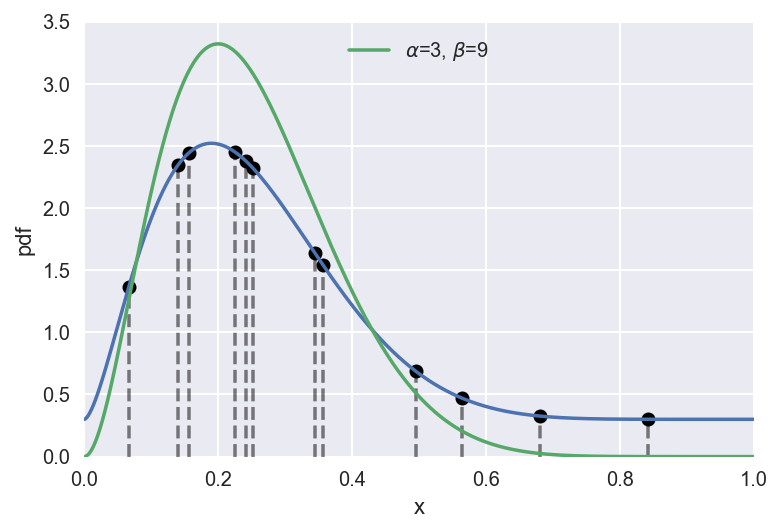The Importance Sampling Estimation is 0.08404


## The Generalized Harmonic Mean Estimator¶

post_samples = st.beta.rvs(k+alpha, n-k+beta, size=N)

# probit-transform posterior samples
probit = st.norm.ppf
probit_post_samples = probit(post_samples)

# correct for change of coordinates, not sure if my derivation is correct
scale = np.log(np.abs((2/np.sqrt(np.pi) * exp(-post_samples**2)).sum()))

mu_probit = np.mean(probit_post_samples)
sd_probit = np.std(probit_post_samples)

sd_g_is = sd_probit/1.5

m_l_gh = 1/(st.norm.pdf(probit_post_samples, mu_probit, sd_g_is)/
(st.binom.pmf(k, n, p=post_samples)*
st.norm.pdf(probit_post_samples, 0, 1))).mean()
print('The Generalized Harmonic Mean is %.5f'%(m_l_gh))

x1 = np.linspace(-2, 1, 1000)

fig, ax = plt.subplots()
betap = st.beta.pdf(post_samples, k+alpha, n-k+beta)
ax.plot(probit_post_samples, betap/scale, 'ko')
ax.plot([probit_post_samples, probit_post_samples],
[np.zeros_like(betap), betap/scale], 'k--', alpha=.5)
ax.plot(x1, st.norm.pdf(x1, mu_probit, sd_g_is))
ax.plot(probit(x), st.beta.pdf(x, k+alpha, n-k+beta)/scale)
plt.legend(loc='upper center', frameon=False)
ax.set(xlim=[-2,1], ylim=[0,2], xlabel='x', ylabel='pdf')
plt.show()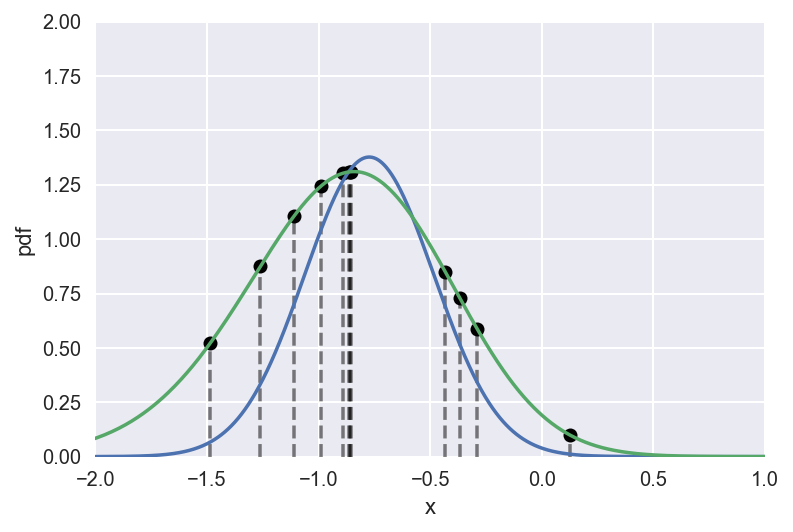The Generalized Harmonic Mean is 0.09226


The posterior sample from PyMC3 is already on a Real line (it transform the paremeters internally for NUTS sampling), we can skip the step of probit transformation.

post_samples = trace['p']
logit_samples = trace['p_logodds__']

mu_probit = np.mean(logit_samples)
sd_probit = np.std(logit_samples)

sd_g_is = sd_probit/2

m_l_gh = 1/(st.norm.pdf(logit_samples, mu_probit, sd_g_is)/
(st.binom.pmf(k, n, p=post_samples)*
st.norm.pdf(logit_samples, 0, 1))).mean()
print('The Generalized Harmonic Mean is %.5f'%(m_l_gh))

The Generalized Harmonic Mean is 0.08860


## The Bridge Sampling Estimator¶

The Bridge Sampling Estimator is a general case of method 1-3 above (more details see Appendix A in the paper). The idea is to build an Importance density using the posterior (for the easy generation of samples), and use a bridge function to "bridge" between the importance samples and the posterior samples.

It goes as the following:

• Draw 2*N1 samples from the $\text{Beta}(3, 9)$ posterior for theta.
N1 = 12
theta_star = st.beta.rvs(k+alpha, n-k+beta, size=N1*2)

• Choose a proposal distribution.
Here using normal distribution as in the paper.
• Transform the first batch of N1 posterior samples. Using a Probit transform here.
theta_star_4_fit = theta_star[:N1]
xi_star_4_fit = probit(theta_star_4_fit)

• Fit the proposal distribution to the first batch of N1 probit-transformed posterior samples.
mu_hat = np.mean(xi_star_4_fit)
sigma_hat = np.std(xi_star_4_fit)

• Draw N2 samples from the proposal distribution.
N2 = N1
xi_tilde = st.norm.rvs(mu_hat, sigma_hat, size=N2)

• Calculate $l_{2,i}$ for all N2 samples from the proposal.
def unnormalized_posterior(xi, k, n):
# returns the unnormalized posterior density for xi
return (st.binom.pmf(k, n, p=st.norm.cdf(xi))*
st.norm.pdf(xi, 0, 1))

l2 = unnormalized_posterior(xi_tilde, k, n)/ \
st.norm.pdf(xi_tilde, mu_hat, sigma_hat)

• Transform the second batch of N1 posterior samples.
theta_star_4_iter = theta_star[N1+1:]
xi_star_4_iter = probit(theta_star_4_iter)

• Calculate $l_{1,j}$ for all N1 probit-transformed samples from the posterior distribution.
l1 = unnormalized_posterior(xi_star_4_iter, k, n)/ \
st.norm.pdf(xi_star_4_iter, mu_hat, sigma_hat)

• Run the iterative scheme until our predefined tolerance criterion is reached.
p4_y = 0 # initial guess of marginal likelihood
tol = 1e-10 # tolerance criterion
criterion_val = tol + 1 # criterion value

s1 = N1/(N1 + N2)
s2 = N2/(N1 + N2)

i = 0 # iteration counter
while criterion_val > tol:
p4_y_old = p4_y
# Equation 15
numerator = l2/(s1*l2 + s2*p4_y)
denominator = 1/(s1*l1 + s2*p4_y)
p4_y = N1/N2*np.sum(numerator)/np.sum(denominator)
i += 1
criterion_val = abs((p4_y - p4_y_old)/p4_y)
print("Iteration: %s -- Marginal likelihood estimate: %.5f"%(i, p4_y))

print('The Bridge Sampling Estimatation is %.5f'%(p4_y))

Iteration: 1 -- Marginal likelihood estimate: 0.05027
Iteration: 2 -- Marginal likelihood estimate: 0.07664
Iteration: 3 -- Marginal likelihood estimate: 0.07899
Iteration: 4 -- Marginal likelihood estimate: 0.07914
Iteration: 5 -- Marginal likelihood estimate: 0.07915
Iteration: 6 -- Marginal likelihood estimate: 0.07915
Iteration: 7 -- Marginal likelihood estimate: 0.07915
Iteration: 8 -- Marginal likelihood estimate: 0.07915
Iteration: 9 -- Marginal likelihood estimate: 0.07915
Iteration: 10 -- Marginal likelihood estimate: 0.07915
Iteration: 11 -- Marginal likelihood estimate: 0.07915
The Bridge Sampling Estimatation is 0.07915


## The Bridge Sampling Estimator in PyMC3¶

There are a few features in PyMC3 eases the implementation of the Bridge Sampling Estimator of the Marginal Likelihood:
1, the log-likelihood function is easily available, and unlike in Stan all the constants are retained so no need to change the way you write down the model;
2, all the parameters are already transformed to have the domain in $\mathbb{R}$, and we can just approximate it with a MvNormal

# Based on https://github.com/quentingronau/bridgesampling/blob/master/R/bridge_sampler_normal.
from pymc3.model import modelcontext
from scipy import dot
from scipy.linalg import cholesky as chol
import warnings

def Marginal_llk(mtrace, model=None, logp=None, maxiter=1000):
"""The Bridge Sampling Estimator of the Marginal Likelihood.

Parameters
----------
mtrace : MultiTrace, result of MCMC run
model : PyMC Model
Optional model. Default None, taken from context.
logp : Model Log-probability function, read from the model by default
maxiter : Maximum number of iterations

Returns
-------
marg_llk : Estimated Marginal log-Likelihood.
"""
r0, tol1, tol2 = 0.5, 1e-10, 1e-4

model = modelcontext(model)
if logp is None:
logp = model.logp_array
vars = model.free_RVs

# Split the samples into two parts
# Use the first 50% for fiting the proposal distribution and the second 50%
# in the iterative scheme.
len_trace = len(mtrace)
nchain = mtrace.nchains

N1_ = len_trace // 2
N1 = N1_*nchain
N2 = len_trace*nchain - N1

neff_list = dict() # effective sample size

arraysz = model.bijection.ordering.size
samples_4_fit = np.zeros((arraysz, N1))
samples_4_iter = np.zeros((arraysz, N2))
# matrix with already transformed samples
for var in vars:
varmap = model.bijection.ordering.by_name[var.name]
# for fitting the proposal
x = mtrace[:N1_][var.name]
samples_4_fit[varmap.slc, :] = x.reshape((x.shape,
np.prod(x.shape[1:], dtype=int))).T
# for the iterative scheme
x2 = mtrace[N1_:][var.name]
samples_4_iter[varmap.slc, :] = x2.reshape((x2.shape,
np.prod(x2.shape[1:], dtype=int))).T
# effective sample size of samples_4_iter, scalar
neff_list.update(pm.effective_n(mtrace[N1_:],varnames=[var.name]))

# median effective sample size (scalar)
neff = pm.stats.dict2pd(neff_list,'temp').median()

# get mean & covariance matrix and generate samples from proposal
m = np.mean(samples_4_fit, axis=1)
V = np.cov(samples_4_fit)
L = chol(V, lower=True)

# Draw N2 samples from the proposal distribution
gen_samples = m[:, None] + dot(L, st.norm.rvs(0, 1,
size=samples_4_iter.shape))

# Evaluate proposal distribution for posterior & generated samples
q12 = st.multivariate_normal.logpdf(samples_4_iter.T, m, V)
q22 = st.multivariate_normal.logpdf(gen_samples.T, m, V)

# Evaluate unnormalized posterior for posterior & generated samples
q11 = np.asarray([logp(point) for point in samples_4_iter.T])
q21 = np.asarray([logp(point) for point in gen_samples.T])

# Iterative scheme as proposed in Meng and Wong (1996) to estimate
# the marginal likelihood
def iterative_scheme(q11, q12, q21, q22, r0, neff, tol, maxiter, criterion):
l1 = q11 - q12
l2 = q21 - q22
lstar = np.median(l1) # To increase numerical stability,
# subtracting the median of l1 from l1 & l2 later
s1 = neff/(neff + N2)
s2 = N2/(neff + N2)
r = r0
r_vals = [r]
logml = np.log(r) + lstar
criterion_val = 1 + tol

i = 0
while (i <= maxiter) & (criterion_val > tol):
rold = r
logmlold = logml
numi = np.exp(l2 - lstar)/(s1 * np.exp(l2 - lstar) + s2 * r)
deni = 1/(s1 * np.exp(l1 - lstar) + s2 * r)
if np.sum(~np.isfinite(numi))+np.sum(~np.isfinite(deni)) > 0:
warnings.warn("""Infinite value in iterative scheme, returning NaN.
Try rerunning with more samples.""")
r = (N1/N2) * np.sum(numi)/np.sum(deni)
r_vals.append(r)
logml = np.log(r) + lstar
i += 1
if criterion=='r':
criterion_val = np.abs((r - rold)/r)
elif criterion=='logml':
criterion_val = np.abs((logml - logmlold)/logml)

if i >= maxiter:
return dict(logml = np.NaN, niter = i, r_vals = np.asarray(r_vals))
else:
return dict(logml = logml, niter = i)

# Run iterative scheme:
tmp = iterative_scheme(q11, q12, q21, q22, r0, neff, tol1, maxiter, 'r')
if ~np.isfinite(tmp['logml']):
warnings.warn("""logml could not be estimated within maxiter, rerunning with
adjusted starting value. Estimate might be more variable than usual.""")
# use geometric mean as starting value
r0_2 = np.sqrt(tmp['r_vals'][-2]*tmp['r_vals'][-1])
tmp = iterative_scheme(q11, q12, q21, q22, r0_2, neff, tol2, maxiter, 'logml')

return dict(logml = tmp['logml'], niter = tmp['niter'], method = "normal",
q11 = q11, q12 = q12, q21 = q21, q22 = q22)


logml_dict = Marginal_llk(trace, model=BB, maxiter=1000)

print('The Bridge Sampling Estimatation is %.5f'%(np.exp(logml_dict['logml'])))

The Bridge Sampling Estimatation is 0.09053


## A more complex example:¶

Based on the bridgesampling R package example.

mu, tau2, sigma2 = 0, 0.5, 1

n = 20
theta = st.norm.rvs(mu, np.sqrt(tau2), size=n)
y = st.norm.rvs(theta, np.sqrt(sigma2), size=n)

# set prior parameters
mu0, tau20 = 0, 1
alpha = beta = 1.


Copying the generated data from R so it is easier to compare...

# Same sample from R, so it is eaiser to compare
y = np.array([1.19365332,  1.95745331, -0.72161754, -1.87380833, -1.16928239,  0.51960853,
-0.03610041,  0.42508815,  0.41119221, -0.81236980,  0.72967357,  3.48186722,
2.31126381,  2.00029422, -0.27643507,  1.06882370, -0.95083599, -1.89651101,
2.56019737,  0.23703060])


Set up the model and sample. You might notice that we are taking a lot of samples (a simple model like this I usually just take 1000 sampples with 2 chains). The reason is that a much larger number of posterior samples is needed to estimate the marginal likelihood.

with pm.Model() as H0:
tau0 = pm.InverseGamma('tau', alpha, beta)
theta0 = pm.Normal('theta', 0, tt.sqrt(tau0), shape=n)
obs0 = pm.Normal('y', theta0, np.sqrt(sigma2), observed=y)
trace0 = pm.sample(10000, tune=1000, njobs=3)

with pm.Model() as H1:
mu1 = pm.Normal('mu', mu0, np.sqrt(tau20))
tau1 = pm.InverseGamma('tau', alpha, beta)
theta1 = pm.Normal('theta', mu1, tt.sqrt(tau1), shape=n)
obs1 = pm.Normal('y', theta1, np.sqrt(sigma2), observed=y)
trace1 = pm.sample(10000, tune=1000, njobs=3)

Auto-assigning NUTS sampler...
100%|██████████| 11000/11000 [00:09<00:00, 1177.08it/s]
Auto-assigning NUTS sampler...
100%|██████████| 11000/11000 [00:10<00:00, 1016.71it/s]


Make sure you check the model fitting - there is no point to go further if the samples are not genuinely from the posterior.

pm.traceplot(trace0);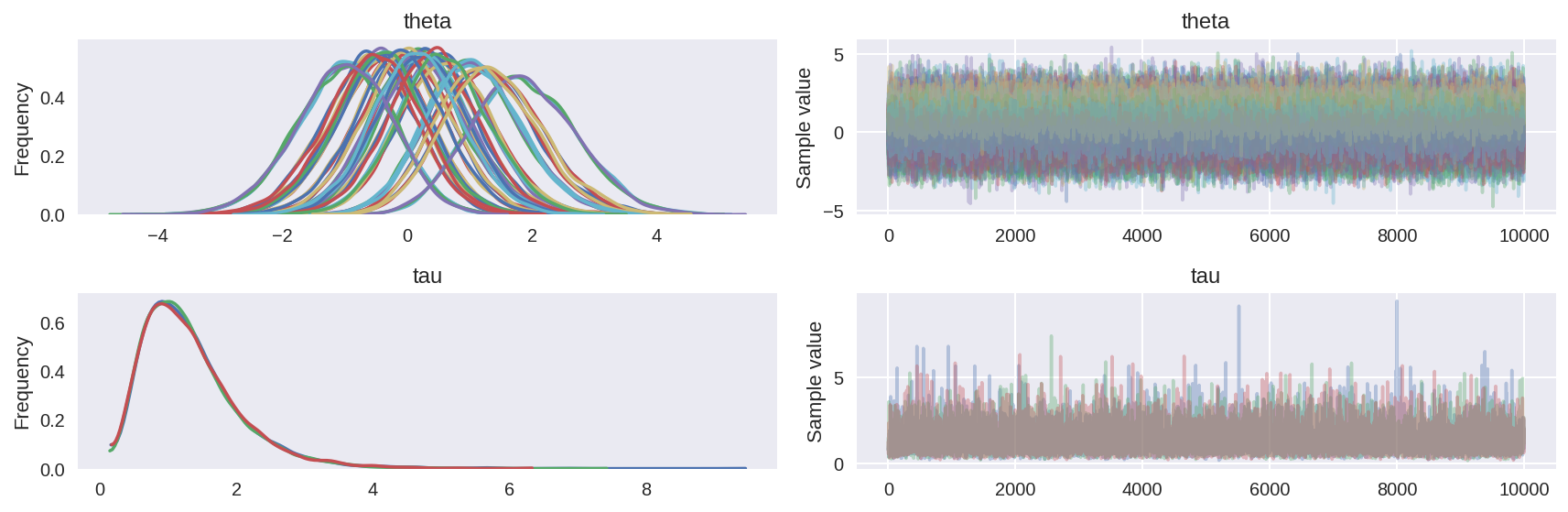logml0 = Marginal_llk(trace0, model=H0, maxiter=1000)
print('Bridge sampling estimate of the log marginal likelihood: %.5f'
%(logml0['logml']))

Bridge sampling estimate of the log marginal likelihood: -37.53850

_, ax=plt.subplots(1,1,figsize=(8,5))
for dist in ['q11', 'q12', 'q21', 'q22']:
sns.distplot(logml0[dist], ax=ax, label=dist)
plt.legend();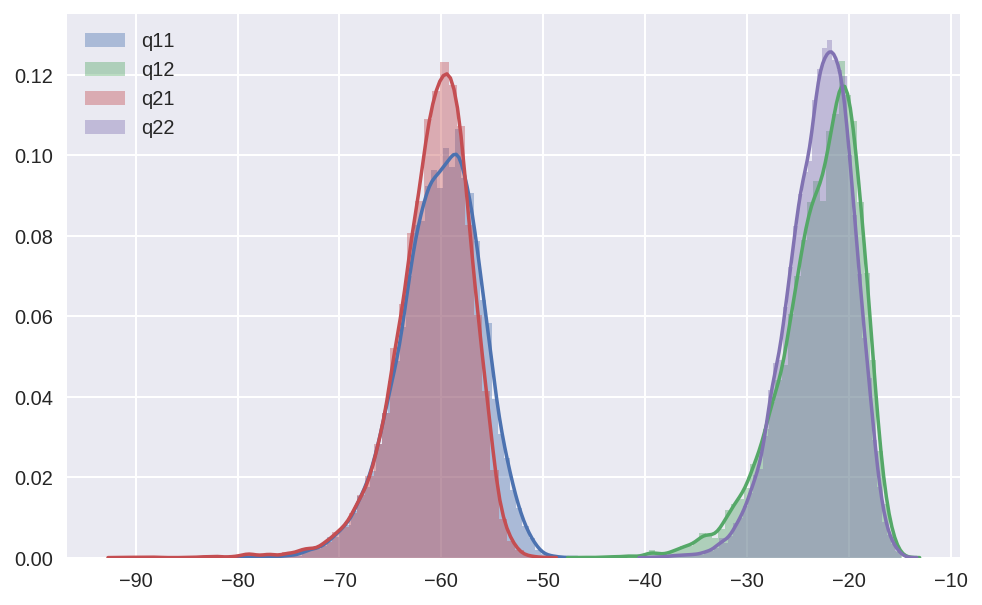The above figure shows:
q11 - the Model log-liklihood of the posterior sample
q12 - the Multivariate Normal log-liklihood of the posterior sample
q21 - the Model log-liklihood of the generated sample
q22 - the Multivariate Normal log-liklihood of the generated sample

With these information, you can then compute the error measures for estimated marginal likelihood. In the R package a function coda::spectrum0.ar is called within the bridgesampling::error_measures, here I wrote a version using AR from statsmodels - result might differ a little bit.

from scipy.linalg import lstsq
from statsmodels.tsa.ar_model import AR
def spectrum0_ar(x):
"""Port of spectrum0.ar from coda::spectrum0.ar"""
z = np.arange(1, len(x)+1)
z = z[:, np.newaxis]**[0, 1]
p, res, rnk, s = lstsq(z, x)
residuals = x - np.matmul(z, p)

if residuals.std() == 0:
spec = order = 0
else:
ar_out = AR(x).fit(ic='aic', trend='c')
order = ar_out.k_ar
spec = np.var(ar_out.resid)/(1 - np.sum(ar_out.params[1:]))**2

return spec, order


def error_measures(logml):
"""Port of the error_measures.R in bridgesampling
https://github.com/quentingronau/bridgesampling/blob/master/R/error_measures.R
"""
ml = np.exp(logml['logml'])
g_p = np.exp(logml['q12'])
g_g = np.exp(logml['q22'])
priorTimesLik_p = np.exp(logml['q11'])
priorTimesLik_g = np.exp(logml['q21'])
p_p = priorTimesLik_p/ml
p_g = priorTimesLik_g/ml

N1 = len(p_p)
N2 = len(g_g)
s1 = N1/(N1 + N2)
s2 = N2/(N1 + N2)

f1 = p_g/(s1*p_g + s2*g_g)
f2 = g_p/(s1*p_p + s2*g_p)
rho_f2, _ = spectrum0_ar(f2)

term1 = 1/N2 * np.var( f1 ) / np.mean( f1 )**2
term2 = rho_f2/N1 * np.var( f2 ) / np.mean( f2 )**2

re2 = term1 + term2

# convert to coefficient of variation (assumes that bridge estimate is unbiased)
cv = np.sqrt(re2)

return dict(re2 = re2, cv = cv)


print('The percentage errors of the estimation is: %.5f'
%(error_measures(logml0)['cv']*100))

The percentage errors of the estimation is: 0.32839 %


(The error is larger than in the R example, because here I am using much less samples.)

And at last, the marginal likelihood for model H1, and also compute the Bayes Factor between the two models.

logml1 = Marginal_llk(trace1, model=H1, maxiter=1000)
print('Bridge sampling estimate of the log marginal likelihood: %.5f'
%(logml1['logml']))

Bridge sampling estimate of the log marginal likelihood: -37.78925

BF01 = np.exp(logml0['logml'] - logml1['logml'])
print('The estimated Bayes factor in favor of Model_1 over Model_2 is equal to: %.5f'
%(BF01))

The estimated Bayes factor in favor of Model_1 over Model_2 is equal to: 1.28499


The result is pretty much the same as in the R example.

## Computing marginal likelihood using SMC¶

There is also another way to compute marginal likelihood in PyMC3, using Sequential Monte Carlo sampler. You can find more information about it in the Documentation.
!Warning!: This can also take a while to sample.

#SMC related set up
from tempfile import mkdtemp
test_folder = mkdtemp(prefix='SMC_TEST')
n_steps = 20
n_chains = 1000

from pymc3.step_methods import smc
with pm.Model() as H0_:
tau0 = pm.InverseGamma('tau', alpha, beta, transform=None)
theta0 = pm.Normal('theta', 0, tt.sqrt(tau0), shape=n)
obs0 = pm.Normal('y', theta0, np.sqrt(sigma2), observed=y)
trace0_ = smc.sample_smc(n_steps=n_steps,
n_chains=n_chains,
progressbar=False,
homepath=test_folder,
stage=0)

with pm.Model() as H1_:
mu1 = pm.Normal('mu', mu0, np.sqrt(tau20))
tau1 = pm.InverseGamma('tau', alpha, beta, transform=None)
theta1 = pm.Normal('theta', mu1, tt.sqrt(tau1), shape=n)
obs1 = pm.Normal('y', theta1, np.sqrt(sigma2), observed=y)
trace1_ = smc.sample_smc(n_steps=n_steps,
n_chains=n_chains,
progressbar=False,
homepath=test_folder,
stage=0)


print('SMC estimate of log marginal likelihood for H0 is: %.5f'
%(np.log(H0_.marginal_likelihood)))
print('SMC estimate of log marginal likelihood for H1 is: %.5f'
%(np.log(H1_.marginal_likelihood)))

SMC estimate of log marginal likelihood for H0 is: -15.20573
SMC estimate of log marginal likelihood for H1 is: -16.49513


BF01_ = H0_.marginal_likelihood / H1_.marginal_likelihood
print('The estimated Bayes factor in favor of Model_1 over Model_2 is equal to: %.5f'
%(BF01_))

The estimated Bayes factor in favor of Model_1 over Model_2 is equal to: 3.63061


The estimated marginal likelihood is not the same as the one estimated using the Bridge sampling, and the Bayes factor is quite different as well. I guess it is time to double check the marginal likelihood estimation in SMC.

References:

• A tutorial on bridge sampling. arXiv preprint arXiv:1703.05984 (2017).
• bridgesampling the R package
• Meng, X.-L., & Wong, W. H. (1996). Simulating ratios of normalizing constants via a simple identity: A theoretical exploration. Statistica Sinica, 6, 831-860.